# Projectile Motion Formulas

Projectile Motion Formulas

A projectile is an object that is given an initial velocity, and is acted on by gravity. The path the object follows is determined by these effects (ignoring air resistance). This path is the object's trajectory. The trajectory has horizontal (x) and vertical (y) components. Velocity is a vector (it has magnitude and direction), so the overall velocity of an object can be found with vector addition of the x and y components: v2 = vx2 + vy2. The units to express the horizontal and vertical distances are meters (m). The horizontal and vertical velocities are expressed in meters per second (m/s).

Horizontal distance

horizontal distance = (initial horizontal velocity)(time)

x = vxo t

Vertical distance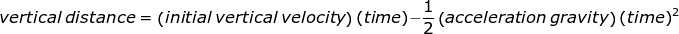Horizontal velocity

horizontal velocity = initial horizontal velocity

vx = vxo

Vertical velocity

vertical velocity = initial vertical velocity - (acceleration due to gravity)(time)

vy = vyo - gt

x = horizontal distance (m)

y = vertical distance (m)

v = velocity (combined components, m/s)

vx = horizontal velocity (m/s)

vy = vertical velocity (m/s)

vxo = initial horizontal velocity (m/s)

vyo = initial vertical velocity (m/s)

t = time (s)

g = acceleration due to gravity (9.80 m/s2)

Projectile Motion Formulas Questions:

1) A child kicks a soccer ball off of the top of a hill. The initial velocity of the ball is 15.0 m/s horizontally. After 5.00 s, what is the magnitude of the velocity of the ball?

Answer: The velocity of the ball after 5.00 s has two components. Once these two components are found, they must be combined using vector addition to find the final velocity. The ball was kicked horizontally, so vxo = 15.0 m/s, and vyo = 0.0 m/s. The x component of the velocity after 5.00 s is:

vx = vxo

and the y component is:

vy = vyo - gt

vy = (0.0 m/s)-(9.80 m/s2)(5.00 s)

vy = -49.0 m/s

In projectile motion problems, up is defined as the positive direction, so the y component has a magnitude of 49.0 m/s, in the down direction.

To find the magnitude of the velocity, the x and y components must be added with vector addition:

v2 = vx2 + vy2

v2 = (15.0 m/s)2 + (-49.0 m/s)2

v2 = 2626 (m/s)2

v = 51.24 m/s

The magnitude of the velocity is 51.24 m/s.

Though it was not asked for in the question, it is also possible to find the direction of the velocity as an angle. If the horizontal direction is 0.0 radians, the angle can be found with the equation: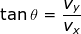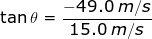tanθ = -3.267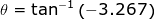θ = -72.98°

Therefore, the velocity (magnitude and direction) of the ball after 5.00 s was 51.24 m/s, -72.98° down from the horizontal.

2) A toy rocket is launched in a flat field, aimed at an angle 60.0° up from the horizontal (x) axis. Its initial velocity has a magnitude of 20.0 m/s. How much time does the toy rocket spend the air, and how far from its launch point does it land in the field?

Answer: The first thing that must be found to solve this problem is the initial velocity in the x and y directions. The velocity that is given has both x and y components, because it is in a direction 60.0° up from the horizontal (x) direction. The initial velocity can be broken down using an equation relating the sine and cosine:

1 = cos2 θ + sin2 θ

We multiply both sides by the initial velocity squared vo2:

(vo2)(1) = (vo2)(cos2 θ) + (vo2)(sin2 θ)

vo2 = (vo cosθ)2 + (vo sinθ)2

The equation for vector addition of the initial velocity components is:

vo2 = vxo2 + vyo2

So, the components of the velocity can be set equal to the parts of the equation above:

vxo2 = (vo cosθ)2

vxo = vo cosθ

and vyo2 = (vo sinθ)2

vyo = vo sinθ

So, the initial velocity in the x direction vxo is:

vxo = vo cosθ

vxo = (20.0 m/s)(cos60°)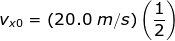vxo = 10.0 m/s

and the initial velocity in the y direction is:

vyo = vo sinθ

vyo = (20.0 m/s)(sin60°)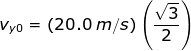vyo = 17.32 m/s

To find how long the toy rocket was in the air, use the equation for the vertical distance for the rocket:The field is flat, so the rocket will hit the ground again at y = 0.00 m. So, set the left side of the equation equal to that height: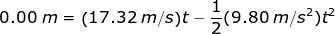(-4.90 m/s2)t2 + (17.32 m/s)t + 0.00 m = 0

This has the form of the quadratic equation, with t as the variable:

If at2 + bt + c = 0,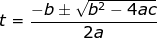.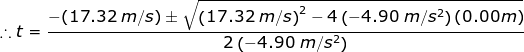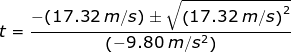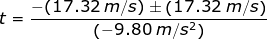There are two solutions for t, depending whether the positive or negative in the ± symbol is chosen. Solving for each: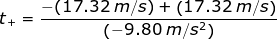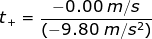t+ = 0.00 s

and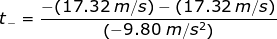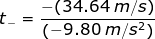t- = 3.53 s

The first solution is the starting time, and the second is the time at which the toy rocket reaches the ground again. Therefore, the amount of time that the toy rocket spent in the air was 3.53 seconds.

To find the distance from the starting position to the landing position, use the equation for horizontal distance:

x = vxo t

The time that the toy rocket traveled through the air was just found to be 3.53 s. Using that number, the horizontal distance is:

x = vxo t

x = (10.0 m/s)(3.53 s)

x = 35.3 m

The toy rocket lands 35.3 m away from its launch position.